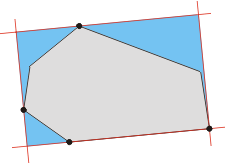• 利用opencv找图像中形状的最小外接矩形，进而可获得最小外接矩形的信息等最小外接矩形
• 凸多边形最小面积外接矩形

千次阅读 2017-11-04 11:24:08
但是我们需要测试每个情形来获得每个矩形来计算最小面积吗？ 谢天谢地， 我们不必那么干。    对于多边形 P 的一个外接矩形存在一条边与原多边形的边共线。    上述结论有力地限制了矩形的可能范围。 我们...

给定一个凸多边形 P ， 面积最小的能装下 P （就外围而言）的矩形是怎样的呢？ 从技术上说， 给定一个方向， 能计算出 P 的端点并且构由此造出外接矩形。 但是我们需要测试每个情形来获得每个矩形来计算最小面积吗？ 谢天谢地， 我们不必那么干。

对于多边形 P 的一个外接矩形存在一条边与原多边形的边共线。

上述结论有力地限制了矩形的可能范围。 我们不仅不必去检测所有可能的方向， 而且只需要检测与多边形边数相等数量的矩形。图示上述结论： 四条切线（红色）， 其中一条与多边形一条边重合， 确定了外接矩形（蓝色）。

一个简单的算法是依次将每条边作为与矩形重合的边进行计算。 但是这种构造矩形的方法涉及到计算多边形每条边端点， 一个花费 O(n) 时间（因为有 n 条边）的计算。 整个算法将有二次时间复杂度。

一个更高效的算法已经发现。 利用旋转卡壳， 我们可以在常数时间内实时更新， 而不是重新计算端点。
实际上， 考虑一个凸多边形， 拥有两对和 x 和 y 方向上四个端点相切的切线。 四条线已经确定了一个多边形的外接矩形。 但是除非多边形有一条水平的或是垂直的边， 这个矩形的面积就不能算入最小面积中。
然而， 可以通过旋转线直到条件满足。 这个过程是下属算法的核心。 假设按照顺时针顺序输入一个凸多边形的n 个顶点。

1. 计算全部四个多边形的端点， 称之为 xminP， xmaxP， yminP， ymaxP
2. 通过四个点构造 P 的四条切线。 他们确定了两个“卡壳”集合。
3. 如果一条（或两条）线与一条边重合， 那么计算由四条线决定的矩形的面积， 并且保存为当前最小值。 否则将当前最小值定义为无穷大。
4. 顺时针旋转线直到其中一条和多边形的一条边重合。
5. 计算新矩形的面积， 并且和当前最小值比较。 如果小于当前最小值则更新， 并保存确定最小值的矩形信息。
6. 重复步骤4和步骤5， 直到线旋转过的角度大于90度。
7. 输出外接矩形的最小面积。

因为两对的“卡壳”确定了一个外接矩形， 这个算法考虑到了所有可能算出最小面积的矩形。 进一步， 除了初始值外， 算法的主循环只需要执行顶点总数多次。 因此算法是线性时间复杂度的。

一个相似但是鲜为人知的问题是最小周长外接矩形问题。 有趣的是这两个问题是完全不同的问题， 因为存在（尽管极少）最小面积外接矩形和最小周长外接矩形多边形不重合的多边形。

展开全文• 矩形面积 Time Limit: 2000/1000 MS (Java/Others) Memory Limit: 32768/32768 K (Java/Others) Total Submission(s): 417 Accepted Submission(s): 239 Problem Description 小度熊有一个桌面，小度熊剪...

矩形面积

Time Limit: 2000/1000 MS (Java/Others)    Memory Limit: 32768/32768 K (Java/Others)
Total Submission(s): 417    Accepted Submission(s): 239

Problem Description
小度熊有一个桌面，小度熊剪了很多矩形放在桌面上，小度熊想知道能把这些矩形包围起来的面积最小的矩形的面积是多少。

Input
第一行一个正整数 T，代表测试数据组数( 1T20)，接下来 T 组测试数据。

每组测试数据占若干行，第一行一个正整数 N(1N<≤1000)，代表矩形的数量。接下来 N 行，每行 8 个整数 x1,y1,x2,y2,x3,y3,x4,y4，代表矩形的四个点坐标，坐标绝对值不会超过10000。

Output
对于每组测试数据，输出两行：

第一行输出"Case #i:"，i 代表第 i 组测试数据。
第二行包含1 个数字，代表面积最小的矩形的面积，结果保留到整数位。

Sample Input

2 2 5 10 5 8 3 10 3 8 8 8 8 6 7 8 7 6 1 0 0 2 2 2 0 0 2

Sample Output

Case #1: 17 Case #2: 4

Source

Recommend
hujie   |   We have carefully selected several similar problems for you:   6010  6009  6008  6007  6006

Statistic |  Submit |  Discuss | Note

题解：旋转卡壳求最小面积外接矩形

先对所以矩形的顶点求凸包，然后考虑求凸包的最小面积外接矩形。

有一点很显然就是外接矩形的某条边上一定包含至少两个凸包上的点，其他边至少包含一个。这样才能确定出一个合法的矩形。

所以我们考虑枚举凸包上的相邻两点，确定矩形的一条边所在的直线。然后就是要求到该直线最高，最左，最右的点。这些点随着凸包上边的旋转是单调的。

最高的好判断，直接用点到直线的距离。

最左最右的话，可以用点积，为什么是点积，因为我们要求的两个点一定在该直线上的投影最长，注意向量的方向。

那么矩形的宽也可以用计算投影的方式统计出来。

#include<iostream>
#include<cstdio>
#include<cstring>
#include<algorithm>
#include<cmath>
#define N 10003
#define eps 1e-7
using namespace std;
struct vector{
double x,y;
vector (double X=0,double Y=0){
x=X,y=Y;
}
}a[N],ch[N];
int n,m,cnt;
double ans;
typedef vector point;
vector operator -(vector a,vector b){
return vector (a.x-b.x,a.y-b.y);
}
vector operator +(vector a,vector b){
return vector (a.x+b.x,a.y+b.y);
}
vector operator *(vector a,double t){
return vector (a.x*t,a.y*t);
}
bool operator <(vector a,vector b){
return a.x<b.x||a.x==b.x&&a.y<b.y;
}
double cross(vector a,vector b){
return a.x*b.y-a.y*b.x;
}
double dot(vector a,vector b){
return a.x*b.x+a.y*b.y;
}
void convexhull()
{
sort(a+1,a+n+1);
if (n==1) {
ch[m++]=a;
return;
}
m=0;
for (int i=1;i<=n;i++){
while (m>1&&cross(ch[m-1]-ch[m-2],a[i]-ch[m-2])<=0) m--;
ch[m++]=a[i];
}
int k=m;
for (int i=n-1;i>=1;i--){
while (m>k&&cross(ch[m-1]-ch[m-2],a[i]-ch[m-2])<=0) m--;
ch[m++]=a[i];
}
m--;
}
int dcmp(double x)
{
if (fabs(x)<eps) return 0;
else return x<0?-1:1;
}
double len(vector a)
{
return sqrt(a.x*a.x+a.y*a.y);
}
double distl(point p,point a,point b)
{
vector v=p-a; vector u=b-a;
return fabs(cross(v,u))/len(u);
}
double calc(point a,point b,point c)
{
double t=dot(a-b,c-a);
t/=len(a-b); t/=len(c-a);
return acos(t);
}
void rotating()
{
ans=1e16;
int i=1,j=1,k=1;
for (int t=2;t<m;t++)
if (distl(ch[t],ch,ch)>=distl(ch[k],ch,ch)||t==2)k=t;
for (int t=2;t<m;t++)
if (dot(ch-ch,ch[t]-ch)>=dot(ch-ch,ch[i]-ch)||t==2)
i=t;
for (int t=2;t<m;t++)
if (dot(ch-ch,ch[t]-ch)>=dot(ch-ch,ch[j]-ch)||t==2) // ch-ch ch[t]-ch 的点积最大，那么投影在向量上的长度就最大。
j=t;
//cout<<dot(ch-ch,ch-ch)<<" "<<dot(ch-ch,ch-ch)<<endl;
//cout<<k<<" "<<i<<" "<<j<<endl;
double h=distl(ch[k],ch,ch);
double l=len(ch-ch)+fabs(dot(ch-ch,ch[i]-ch))/len(ch-ch)+fabs(dot(ch-ch,ch[j]-ch))/len(ch-ch);
ans=min(ans,h*l);
ch[m]=ch;
for (int t=1;t<m;t++){
while (distl(ch[k],ch[t],ch[t+1])<=distl(ch[(k+1)%m],ch[t],ch[t+1]))
k=(k+1)%m;
if (i==t+1) i=(i+1)%m;
while (dot(ch[t+1]-ch[t],ch[i]-ch[t+1])<=dot(ch[t+1]-ch[t],ch[(i+1)%m]-ch[t+1]))
i=(i+1)%m;
while (dot(ch[t]-ch[t+1],ch[j]-ch[t])<=dot(ch[t]-ch[t+1],ch[(j+1)%m]-ch[t]))
j=(j+1)%m;
if (j==t) j=((j-1)%m+m)%m;
h=distl(ch[k],ch[t],ch[t+1]);
l=len(ch[t]-ch[t+1])+fabs(dot(ch[t+1]-ch[t],ch[i]-ch[t+1]))/len(ch[t]-ch[t+1])+fabs(dot(ch[t]-ch[t+1],ch[j]-ch[t]))/len(ch[t]-ch[t+1]);
ans=min(ans,h*l);
}
}
int main()
{
freopen("a.in","r",stdin);
freopen("my.out","w",stdout);
int t; scanf("%d",&t);
for (int T=1;T<=t;T++){
scanf("%d",&n); cnt=0;
for (int i=1;i<=n;i++){
for (int j=0;j<4;j++)
++cnt,scanf("%lf%lf",&a[cnt].x,&a[cnt].y);
}
n=cnt;
convexhull();
//for (int i=0;i<m;i++) cout<<ch[i].x<<" "<<ch[i].y<<endl;
rotating();
printf("Case #%d:\n",T);
//printf("%.4lf\n",ans);
printf("%.0lf\n",ans);
}
}

展开全文• 但是我们需要测试每个情形来获得每个矩形来计算最小面积吗？ 谢天谢地， 我们不必那么干。 对于多边形 P 的一个外接矩形存在一条边与原多边形的边共线。 上述结论有力地限制了矩形的可能范围。 我们不仅不必去...
给定一个凸多边形 P ， 面积最小的能装下 P （就外围而言）的矩形是怎样的呢？ 从技术上说， 给定一个方向， 能计算出 P 的端点并且构由此造出外接矩形。 但是我们需要测试每个情形来获得每个矩形来计算最小面积吗？ 谢天谢地， 我们不必那么干。

对于多边形 P 的一个外接矩形存在一条边与原多边形的边共线。

上述结论有力地限制了矩形的可能范围。 我们不仅不必去检测所有可能的方向， 而且只需要检测与多边形边数相等数量的矩形。图示上述结论： 四条切线（红色）， 其中一条与多边形一条边重合， 确定了外接矩形（蓝色）。

一个简单的算法是依次将每条边作为与矩形重合的边进行计算。 但是这种构造矩形的方法涉及到计算多边形每条边端点， 一个花费 O(n) 时间（因为有 n 条边）的计算。 整个算法将有二次时间复杂度。

一个更高效的算法已经发现。 利用旋转卡壳， 我们可以在常数时间内实时更新， 而不是重新计算端点。
实际上， 考虑一个凸多边形， 拥有两对和 x 和 y 方向上四个端点相切的切线。 四条线已经确定了一个多边形的外接矩形。 但是除非多边形有一条水平的或是垂直的边， 这个矩形的面积就不能算入最小面积中。
然而， 可以通过旋转线直到条件满足。 这个过程是下属算法的核心。 假设按照顺时针顺序输入一个凸多边形的n 个顶点。

1. 计算全部四个多边形的端点， 称之为 xminP， xmaxP， yminP， ymaxP
2. 通过四个点构造 P 的四条切线。 他们确定了两个“卡壳”集合。
3. 如果一条（或两条）线与一条边重合， 那么计算由四条线决定的矩形的面积， 并且保存为当前最小值。 否则将当前最小值定义为无穷大。
4. 顺时针旋转线直到其中一条和多边形的一条边重合。
5. 计算新矩形的面积， 并且和当前最小值比较。 如果小于当前最小值则更新， 并保存确定最小值的矩形信息。
6. 重复步骤4和步骤5， 直到线旋转过的角度大于90度。
7. 输出外接矩形的最小面积。

因为两对的“卡壳”确定了一个外接矩形， 这个算法考虑到了所有可能算出最小面积的矩形。 进一步， 除了初始值外， 算法的主循环只需要执行顶点总数多次。 因此算法是线性时间复杂度的。

一个相似但是鲜为人知的问题是最小周长外接矩形问题。 有趣的是这两个问题是完全不同的问题， 因为存在（尽管极少）最小面积外接矩形和最小周长外接矩形多边形不重合的多边形。

展开全文算法 python 机器学习 java 数据结构
• 主要为大家详细介绍了Opencv绘制最小外接矩形最小外接圆的方法，具有一定的参考价值，感兴趣的小伙伴们可以参考一下
• 求点集的最小面积外接矩形 void Rotate(float& x, float& y, float angle) { float a = x * cos(angle) - y * sin(angle); float b = x * sin(angle) + y * cos(angle); x = a; y = b; } void Rect...

求点集的最小面积外接矩形

void Rotate(float& x, float& y, float angle) {
float a = x * cos(angle) - y * sin(angle);
float b = x * sin(angle) + y * cos(angle);
x = a;
y = b;
}

void Rect(const vector<float>& x,
const vector<float>& y,
float& length,
float& width) {
float area = 1024;
int size = x.size();
// rotate 0~90 degree
for (int i = 0; i < 91; ++i) {
float tmpx = x, tmpy = y;
Rotate(tmpx, tmpy, i);
float xl = tmpx, xr = tmpx, yt = tmpy, yb = tmpy;
// traverse all points
for (int j = 1; j < size; ++j) {
tmpx = x[j];
tmpy = y[j];
Rotate(tmpx, tmpy, i);
if (tmpx < xl)
xl = tmpx;
if (tmpx > xr)
xr = tmpx;
if (tmpy < yb)
yb = tmpy;
if (tmpy > yt)
yt = tmpy;
}
float xx = xr - xl, yy = yt - yb;
if (area > xx * yy) {
area = xx * yy;
length = xx;
width = yy;
}
}
//
if (length < width) {
float tmp = length;
length = width;
width = tmp;
}
}

展开全文• 简单多边形的最小外接矩形算法，适用于玻璃排样等。
• 凸多边形最小面积外接矩形 给定一个凸多边形 P ， 面积最小的能装下 P （就外围而言）的矩形是怎样的呢？ 从技术上说， 给定一个方向， 能计算出 P 的端点并且构由此造出外接矩形。 但是我们需要测试每个...
• 【凸多边形间最小距离】POJ - 3608 - Bridge Across Islands 题目链接 http://poj.org/problem?id=3608 # include # include # include # include # include using namespace std ; ...旋转卡壳
• 最小外接矩形面积（C++实现）求最小外接矩形的原理C++实现代码 求最小外接矩形的原理 最近在做课程设计的过程中遇到了一个问题，需要求出一幅图像中物体的矩形度，但是求解矩形度需要用到该物体的最小外接矩形的...c++ 图像处理
• 最小外接矩形

2018-01-27 21:55:47
凸包 蛮力 最小面积 最小外接矩形 文件选择 随机生成 凸包点位 然后求解
• 包含多个区域的最小外接矩形''' image = cv2.imread('./label.png') B, G, R = cv2.split(image) ret, thresh = cv2.threshold(G, 128, 255, cv2.THRESH_BINARY) print(thresh.shape) # 单通道复制为三通道 ...代替...
• 主要介绍了Python实现图片查找轮廓、多边形拟合、最小外接矩形代码，具有很好的参考价值，希望对大家有所帮助。一起跟随小编过来看看吧
• 使用opencv画出图形的最小外接矩形最小外接圆，首先求出图形的轮廓，设有滚动条可以选择最佳阈值，然后画出图形的最小外接圆与最小外接矩形，算法的效果很好！
• 题目链接：...题意：求给定点集的最小面积外接矩形。 思路：模板(见我博客内)。 #include #include #include #include #define min(x,y) (旋转卡壳 最小面积外接矩形 计算几何
• matlab计算目标最小外接矩形,主要利用minboundrect函数。
• 本代码是本人亲自写的，描写了一个如何把点放在一个矩形面积下使得矩形面积最小代码
• 今天做UVA 10173时遇到的问题，网上各位大牛的代码和思想实在无法理解，请教学长后理解了一个算法。 ... 下面贴一下学长的模板，图和注释是我加上的。...注：浅褐色为凸包，红色为待求矩形。 #include #include #i凸包 最小面积外接矩形 计算几何
• 主要介绍了python3+openCV 获取图片中文本区域的最小外接矩形实例，具有很好的参考价值，希望对大家有所帮助。一起跟随小编过来看看吧
• 最小外接矩形(MBR)

万次阅读 2014-07-27 10:36:34
最小外接矩形(MBR)可分为最小面积外接矩形(Minimum Area Bounding Rectangle,简称 MABR)和最小周长外接矩形(Minimum Perimter Bounding Rectangle, 简称MPBR)。通常情况下MABR与MPBR差异不大。 图像中物体...计算机视觉 二值化 最小外接矩形 模式识别
• 求解最小外接矩形

千次阅读 2018-12-27 14:15:43
給出一个平面点集S，求一个面积最小矩形使其包含S所有的点。 预备知识：  在求解这道题之前我们先要了解一些关于凸包的知识。  什么是凸包？简单地说，对于一个平面点集S，我们把完全包含该...内接矩形 外接矩形
• 使用python opencv返回点集cnt的最小外接矩形，所用函数为 cv2.minAreaRect(cnt) ，cnt是点集数组或向量（里面存放的是点的坐标），并且这个点集不定个数。 举例说明：画一个任意四边形（任意多边形都可以）的最小...
• 实现提取图像的指定区域的最小外接矩形。 代码： Halcon实现： read_image (M1, 'C:/Users/15383/Desktop/insert/m1.jpg') dev_close_window() dev_open_window(0, 0, 512, 512, 'black', WindowHandle) dev_...
• nbsp图形图像一种获取图像区域最小外接矩形的算法及实现.pdf3页本文档一共被下载：次,您可全文免费在线阅读后下载本文档。 下载提示1.本站不保证该用户上传的文档完整性，不预览、不比对内容而直接下载产生的反悔...
• opencv 获取最小外接矩形

千次阅读 2019-05-19 16:21:31
//最小外接矩形的4个端点 width = box[i].size.width; height = box[i].size.height; if (height >= width) { float x = 0; x = height; height = width; width = x; } ratio = width / ...最小外接矩形 opencv c++...